The PLS Procedure

## Example 66.1 Examining Model Details

This example, from Umetrics (1995), demonstrates different ways to examine a PLS model. The data come from the field of drug discovery. New drugs are developed from chemicals that are biologically active. Testing a compound for biological activity is an expensive procedure, so it is useful to be able to predict biological activity from cheaper chemical measurements. In fact, computational chemistry makes it possible to calculate certain chemical measurements without even making the compound. These measurements include size, lipophilicity, and polarity at various sites on the molecule. The following statements create a data set named pentaTrain, which contains these data.

```   data pentaTrain;
input obsnam \$ S1 L1 P1 S2 L2 P2
S3 L3 P3 S4 L4 P4
S5 L5 P5  log_RAI @@;
n = _n_;
datalines;
VESSK    -2.6931 -2.5271 -1.2871  3.0777  0.3891 -0.0701
1.9607 -1.6324  0.5746  1.9607 -1.6324  0.5746
2.8369  1.4092 -3.1398                    0.00
VESAK    -2.6931 -2.5271 -1.2871  3.0777  0.3891 -0.0701
1.9607 -1.6324  0.5746  0.0744 -1.7333  0.0902
2.8369  1.4092 -3.1398                    0.28
VEASK    -2.6931 -2.5271 -1.2871  3.0777  0.3891 -0.0701
0.0744 -1.7333  0.0902  1.9607 -1.6324  0.5746
2.8369  1.4092 -3.1398                    0.20
VEAAK    -2.6931 -2.5271 -1.2871  3.0777  0.3891 -0.0701
0.0744 -1.7333  0.0902  0.0744 -1.7333  0.0902
2.8369  1.4092 -3.1398                    0.51
VKAAK    -2.6931 -2.5271 -1.2871  2.8369  1.4092 -3.1398
0.0744 -1.7333  0.0902  0.0744 -1.7333  0.0902
2.8369  1.4092 -3.1398                    0.11
VEWAK    -2.6931 -2.5271 -1.2871  3.0777  0.3891 -0.0701
-4.7548  3.6521  0.8524  0.0744 -1.7333  0.0902
2.8369  1.4092 -3.1398                    2.73
VEAAP    -2.6931 -2.5271 -1.2871  3.0777  0.3891 -0.0701
0.0744 -1.7333  0.0902  0.0744 -1.7333  0.0902
-1.2201  0.8829  2.2253                    0.18
VEHAK    -2.6931 -2.5271 -1.2871  3.0777  0.3891 -0.0701
2.4064  1.7438  1.1057  0.0744 -1.7333  0.0902
2.8369  1.4092 -3.1398                    1.53
VAAAK    -2.6931 -2.5271 -1.2871  0.0744 -1.7333  0.0902
0.0744 -1.7333  0.0902  0.0744 -1.7333  0.0902
2.8369  1.4092 -3.1398                   -0.10
GEAAK     2.2261 -5.3648  0.3049  3.0777  0.3891 -0.0701
0.0744 -1.7333  0.0902  0.0744 -1.7333  0.0902
2.8369  1.4092 -3.1398                   -0.52
LEAAK    -4.1921 -1.0285 -0.9801  3.0777  0.3891 -0.0701
0.0744 -1.7333  0.0902  0.0744 -1.7333  0.0902
2.8369  1.4092 -3.1398                    0.40
FEAAK    -4.9217  1.2977  0.4473  3.0777  0.3891 -0.0701
0.0744 -1.7333  0.0902  0.0744 -1.7333  0.0902
2.8369  1.4092 -3.1398                    0.30
VEGGK    -2.6931 -2.5271 -1.2871  3.0777  0.3891 -0.0701
2.2261 -5.3648  0.3049  2.2261 -5.3648  0.3049
2.8369  1.4092 -3.1398                   -1.00
VEFAK    -2.6931 -2.5271 -1.2871  3.0777  0.3891 -0.0701
-4.9217  1.2977  0.4473  0.0744 -1.7333  0.0902
2.8369  1.4092 -3.1398                    1.57
VELAK    -2.6931 -2.5271 -1.2871  3.0777  0.3891 -0.0701
-4.1921 -1.0285 -0.9801  0.0744 -1.7333  0.0902
2.8369  1.4092 -3.1398                    0.59
;
```

You would like to study the relationship between these measurements and the activity of the compound, represented by the logarithm of the relative Bradykinin activating activity (log_RAI). Notice that these data consist of many predictors relative to the number of observations. Partial least squares is especially appropriate in this situation as a useful tool for finding a few underlying predictive factors that account for most of the variation in the response. Typically, the model is fit for part of the data (the "training" or "work" set), and the quality of the fit is judged by how well it predicts the other part of the data (the "test" or "prediction" set). For this example, the first 15 observations serve as the training set and the rest constitute the test set (refer to Ufkes et al. 1978; Ufkes et al. 1982).

When you fit a PLS model, you hope to find a few PLS factors that explain most of the variation in both predictors and responses. Factors that explain response variation provide good predictive models for new responses, and factors that explain predictor variation are well represented by the observed values of the predictors. The following statements fit a PLS model with two factors and save predicted values, residuals, and other information for each data point in a data set named outpls.

```   proc pls data=pentaTrain;
model log_RAI = S1-S5 L1-L5 P1-P5;
run;
```

The PLS procedure displays a table, shown in Output 66.1.1, showing how much predictor and response variation is explained by each PLS factor.

Output 66.1.1 Amount of Training Set Variation Explained
The PLS Procedure

Percent Variation Accounted for by Partial
Least Squares Factors
Number of
Extracted
Factors
Model Effects Dependent Variables
Current Total Current Total
1 16.9014 16.9014 89.6399 89.6399
2 12.7721 29.6735 7.8368 97.4767
3 14.6554 44.3289 0.4636 97.9403
4 11.8421 56.1710 0.2485 98.1889
5 10.5894 66.7605 0.1494 98.3383
6 5.1876 71.9481 0.2617 98.6001
7 6.1873 78.1354 0.2428 98.8428
8 7.2252 85.3606 0.1926 99.0354
9 6.7285 92.0891 0.0725 99.1080
10 7.9076 99.9967 0.0000 99.1080
11 0.0033 100.0000 0.0099 99.1179
12 0.0000 100.0000 0.0000 99.1179
13 0.0000 100.0000 0.0000 99.1179
14 0.0000 100.0000 0.0000 99.1179
15 0.0000 100.0000 0.0000 99.1179

From Output 66.1.1, note that 97% of the response variation is already explained by just two factors, but only 29% of the predictor variation is explained.

The graphics in PROC PLS, available when ODS Graphics is in effect, make it easier to see features of the PLS model.

If you enable ODS Graphics, then in addition to the tables discussed previously, PROC PLS displays a graphical depiction of the R-square analysis as well as a correlation loadings plot summarizing the model based on the first two PLS factors. The following statements perform the previous analysis with ODS Graphics enabled, producing Output 66.1.2 and Output 66.1.3.

```   ods graphics on;

proc pls data=pentaTrain;
model log_RAI = S1-S5 L1-L5 P1-P5;
run;

ods graphics off;
```

Output 66.1.2 Plot of Proportion of Variation Accounted For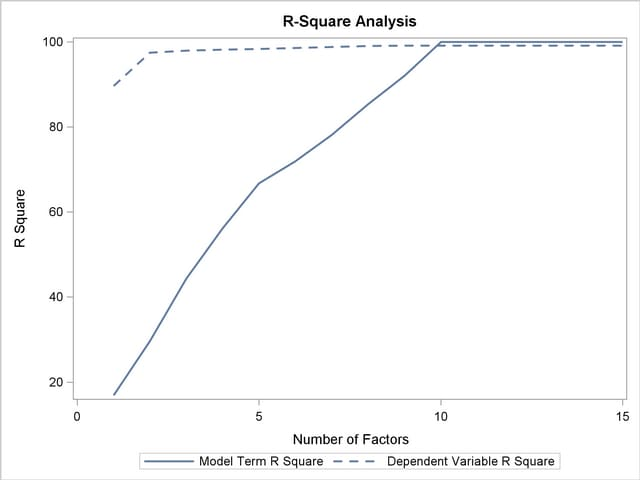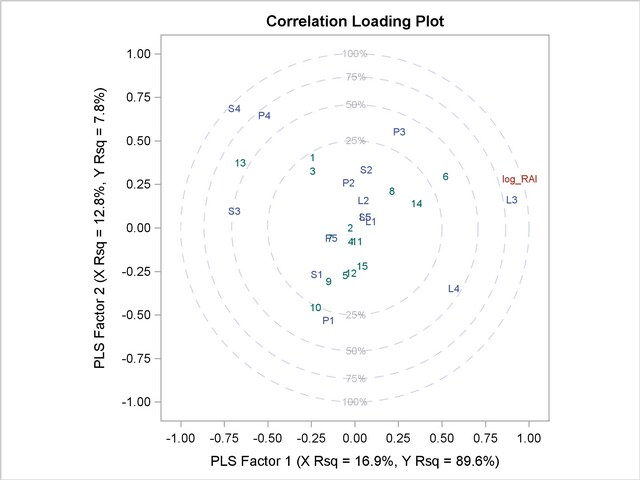The plot in Output 66.1.2 of the proportion of variation explained (or R square) makes it clear that there is a plateau in the response variation after two factors are included in the model. The correlation loading plot in Output 66.1.3 summarizes many features of this two-factor model, including the following:

• The X-scores are plotted as numbers for each observation. You should look for patterns or clearly grouped observations. If you see a curved pattern, for example, you might want to add a quadratic term. Two or more groupings of observations indicate that it might be better to analyze the groups separately, perhaps by including classification effects in the model. This plot appears to show most of the observations close together, with a few being more spread out with larger positive X-scores for factor 2. There are no clear grouping patterns, but observation 13 stands out.

• The loadings show how much variation in each variable is accounted for by the first two factors, jointly by the distance of the corresponding point from the origin and individually by the distance for the projections of this point onto the horizontal and vertical axes. That the dependent variable is well explained by the model is reflected in the fact that the point for log_RAI is near the 100% circle.

• You can also use the projection interpretation to relate variables to each other. For example, projecting other variables’ points onto the line that runs through the log_RAI point and the origin, you can see that the PLS approximation for the predictor L3 is highly positively correlated with log_RAI, S3 is somewhat less correlated but in the negative direction, and several predictors including L1, L5, and S5 have very little correlation with log_RAI.

Other graphics enable you to explore more of the features of the PLS model. For example, you can examine the X-scores versus the Y-scores to explore how partial least squares chooses successive factors. For a good PLS model, the first few factors show a high correlation between the X- and Y-scores. The correlation usually decreases from one factor to the next. When ODS Graphics is in effect, you can plot the X-scores versus the Y-scores by using the PLOT=XYSCORES option, as shown in the following statements.

```   ods graphics on;

proc pls data=pentaTrain nfac=4 plot=XYScores;
model log_RAI = S1-S5 L1-L5 P1-P5;
run;

ods graphics off;
```

The plot of the X-scores versus the Y-scores for the first four factors is shown in Output 66.1.4.

Output 66.1.4 X-Scores versus Y-Scores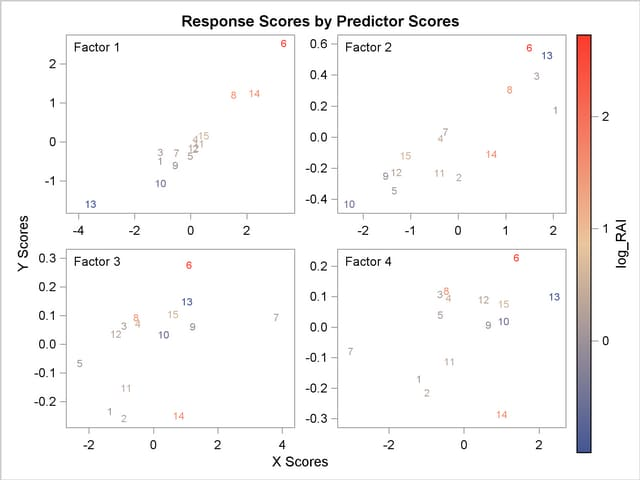For this example, Output 66.1.4 shows high correlation between X- and Y-scores for the first factor but somewhat lower correlation for the second factor and sharply diminishing correlation after that. This adds strength to the judgment that NFAC=2 is the right number of factors for these data and this model. Note that observation 13 is again extreme in the first two plots. This run might be overly influential for the PLS analysis; thus, you should check to make sure it is reliable.

As explained earlier, you can draw some inferences about the relationship between individual predictors and the dependent variable from the correlation loading plot. However, the regression coefficient profile and the variable importance plot give a more direct indication of which predictors are most useful for predicting the dependent variable. The regression coefficients represent the importance each predictor has in the prediction of just the response. The variable importance plot, on the other hand, represents the contribution of each predictor in fitting the PLS model for both predictors and response. It is based on the Variable Importance for Projection (VIP) statistic of Wold (1994), which summarizes the contribution a variable makes to the model. If a predictor has a relatively small coefficient (in absolute value) and a small value of VIP, then it is a prime candidate for deletion. Wold in Umetrics (1995) considers a value less than 0.8 to be "small" for the VIP. The following statements fit a two-factor PLS model and display these two additional plots.

```   ods graphics on;

proc pls data=pentaTrain nfac=2 plot=(ParmProfiles VIP);
model log_RAI = S1-S5 L1-L5 P1-P5;
run;

ods graphics off;
```

The additional graphics are shown in Output 66.1.5 and Output 66.1.6.

Output 66.1.5 Variable Importance Plots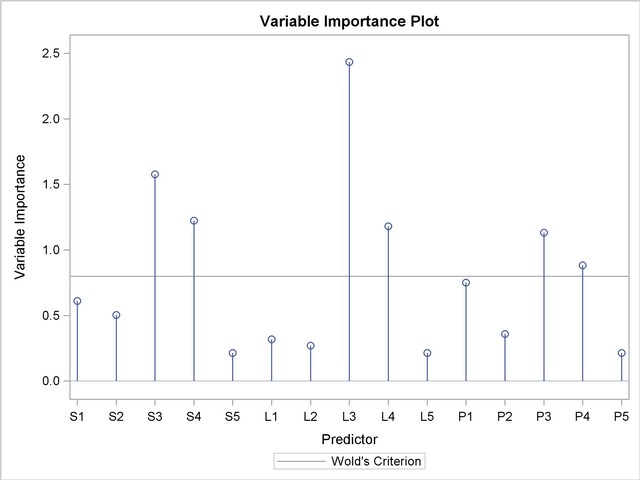Output 66.1.6 Regression Parameter Profile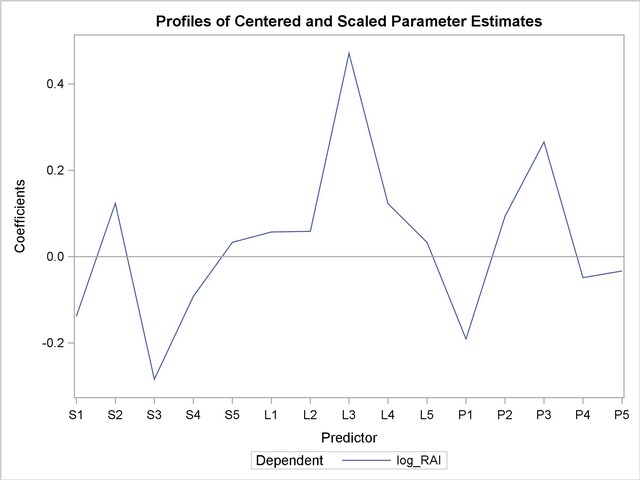In these two plots, the variables L1, L2, P2, S5, L5, and P5 have small absolute coefficients and small VIP. Looking back at the correlation loadings plot in Output 66.1.2, you can see that these variables tend to be the ones near zero for both PLS factors. You should consider dropping these variables from the model.Previous Page | Next Page | Top of Page